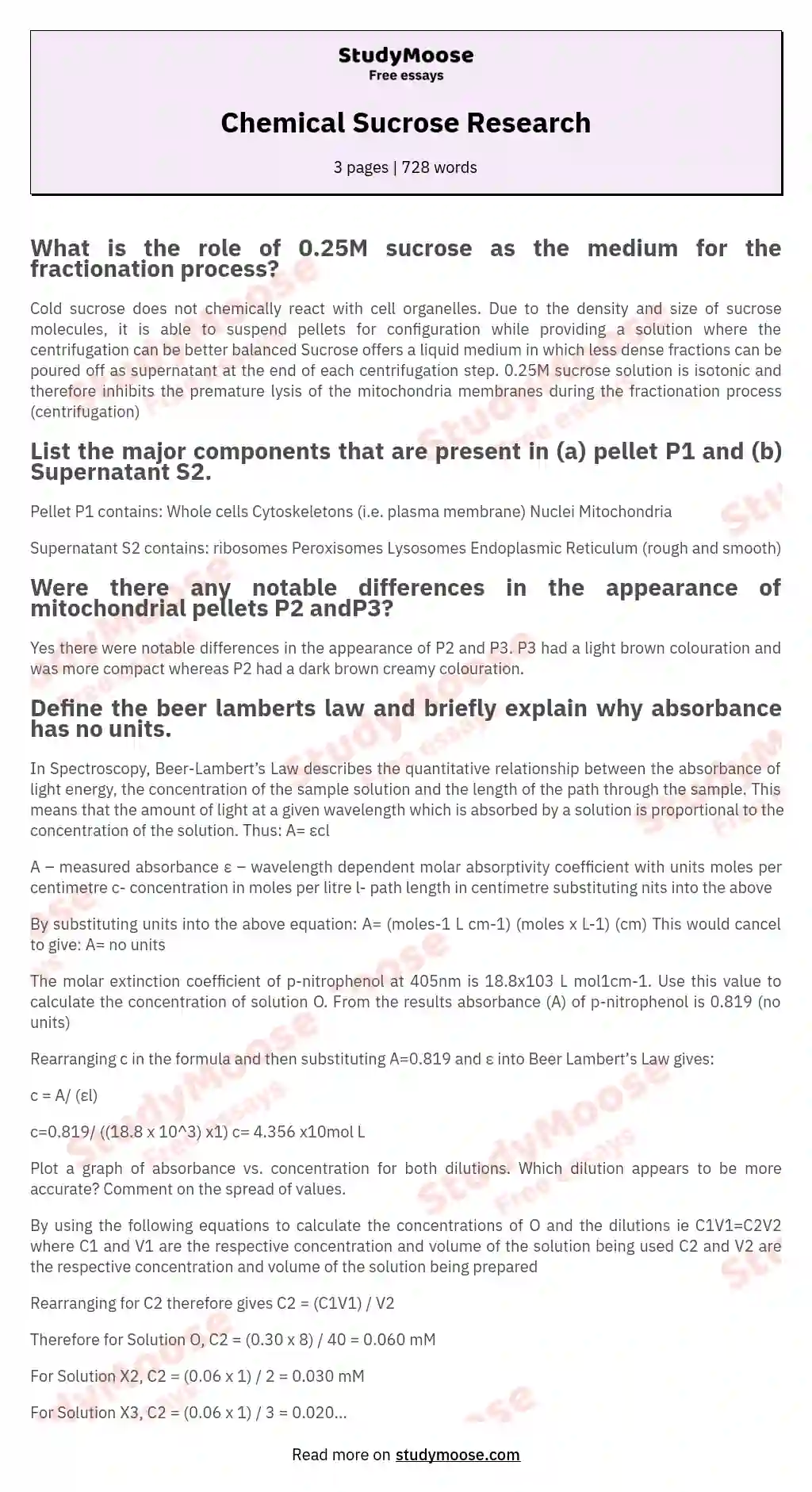# Chemical Sucrose Research

Categories: PhysicsResearch

## What is the role of 0.25M sucrose as the medium for the fractionation process?

Cold sucrose does not chemically react with cell organelles. Due to the density and size of sucrose molecules, it is able to suspend pellets for configuration while providing a solution where the centrifugation can be better balanced Sucrose offers a liquid medium in which less dense fractions can be poured off as supernatant at the end of each centrifugation step. 0.25M sucrose solution is isotonic and therefore inhibits the premature lysis of the mitochondria membranes during the fractionation process (centrifugation)

## List the major components that are present in (a) pellet P1 and (b) Supernatant S2. Don't use plagiarized sources. Get your custom essay on “ Chemical Sucrose Research ” NEW! smart matching with writer

Pellet P1 contains: Whole cells
Cytoskeletons (i.e. plasma membrane) Nuclei
Mitochondria

Supernatant S2 contains: ribosomes
Peroxisomes
Lysosomes
Endoplasmic Reticulum (rough and smooth)

## Were there any notable differences in the appearance of mitochondrial pellets P2 andP3?

Yes there were notable differences in the appearance of P2 and P3. P3 had a light brown colouration and was more compact whereas P2 had a dark brown creamy colouration.

Get quality help nowDr. Karlyna PhDVerified writer

Proficient in: Physics4.7 (235)

“ Amazing writer! I am really satisfied with her work. An excellent price as well. ”+84 relevant experts are online

## Define the beer lamberts law and briefly explain why absorbance has no units.

In Spectroscopy, Beer-Lambert’s Law describes the quantitative relationship between the absorbance of light energy, the concentration of the sample solution and the length of the path through the sample. This means that the amount of light at a given wavelength which is absorbed by a solution is proportional to the concentration of the solution. Thus: A= εcl

A – measured absorbance
ε – wavelength dependent molar absorptivity coefficient with units moles per centimetre c- concentration in moles per litre
l- path length in centimetre substituting nits into the above

By substituting units into the above equation:
A= (moles-1 L cm-1) (moles x L-1) (cm)
This would cancel to give: A= no units

The molar extinction coefficient of p-nitrophenol at 405nm is 18.

Get to Know The Price Estimate For Your Paper
Topic
Number of pages
Email Invalid email

You won’t be charged yet!

8x103 L mol1cm-1. Use this value to calculate the concentration of solution O.
From the results absorbance (A) of p-nitrophenol is 0.819 (no units)

Rearranging c in the formula and then substituting A=0.819 and ε into Beer Lambert’s Law gives:

c = A/ (εl)

c=0.819/ ((18.8 x 10^3) x1)
c= 4.356 x10mol L

Plot a graph of absorbance vs. concentration for both dilutions. Which dilution appears to be more accurate? Comment on the spread of values.

By using the following equations to calculate the concentrations of O and the dilutions
ie C1V1=C2V2
where C1 and V1 are the respective concentration and volume of the solution being used C2 and V2 are the respective concentration and volume of the solution being prepared

Rearranging for C2 therefore gives
C2 = (C1V1) / V2

Therefore for Solution O,
C2 = (0.30 x 8) / 40 = 0.060 mM

For Solution X2,
C2 = (0.06 x 1) / 2 = 0.030 mM

For Solution X3,
C2 = (0.06 x 1) / 3 = 0.020 mM

For Solution X4,
C2 = (0.06 x 1) / 4 = 0.015 mM

For Solution X5,
C2 = (0.06 x 1) / 5 = 0.012 mM

From both graphs, a general direct proportional relationship between absorbance and concentration was observed, where an increase in concentration increased the absorbance. This was deduced based on the linear plot. From comparing both graphs, one clear observation can be seen with the deviation of values from the best-fit line where the values obtained using the automatic pipette had less deviation from the best-fit than those obtained using the measuring cylinder. Hence the dilutions made via the automatic pipette were more accurate. Also, on noting calibration errors in the instruments, the automatic pipette had a relatively small error of ±0.005ml while the measuring cylinder had a larger error of ±0.05ml

Tabulate the data for the pH measurements. State whether your test solution did or did not exhibit buffering capacity. Explain your observation based on the composition of the solution.

Would not make a good buffer as addition of acid lowered the pH by 1.81 while addition of base lowered the pH by 1.63 C

Would make a good buffering solution as addition of acid only changed the pH by 0.28 while addition of base changed the pH by 0.11. Note that sodium hydroxide lowered the pH in tube 4 and this could have been due to experimenter’s error D

Would make a fairly good buffering solution as addition of acid changed the pH by 0.33 while the addition of base changed the pH by 0.46 Table 2 showing solutions A to D and the corresponding inference.

List the major buffer systems in the blood of mammals
Such major systems are

The Carbonic Acid-Bicarbonate buffer

Phosphate buffer

Haemoglobin buffer